Courses

# Cache Memory - MCQ Quiz - 1

## 30 Questions MCQ Test RRB JE for Computer Science Engineering | Cache Memory - MCQ Quiz - 1

Description
This mock test of Cache Memory - MCQ Quiz - 1 for Computer Science Engineering (CSE) helps you for every Computer Science Engineering (CSE) entrance exam. This contains 30 Multiple Choice Questions for Computer Science Engineering (CSE) Cache Memory - MCQ Quiz - 1 (mcq) to study with solutions a complete question bank. The solved questions answers in this Cache Memory - MCQ Quiz - 1 quiz give you a good mix of easy questions and tough questions. Computer Science Engineering (CSE) students definitely take this Cache Memory - MCQ Quiz - 1 exercise for a better result in the exam. You can find other Cache Memory - MCQ Quiz - 1 extra questions, long questions & short questions for Computer Science Engineering (CSE) on EduRev as well by searching above.
QUESTION: 1

### A cache line is 64 bytes. The main memory has latency 32ns and bandwidth 1G.Bytes/s. The time required to fetch theentire cache line from the main memory is

Solution:

ans : c
for 1 sec it is 10^9 bytes so for 64 bytes?
it is 64 * 1 /10^9 so it is 64 ns but mm latency is 32 so total time required to place cache line is 64+32 = 96 ns

QUESTION: 2

### A computer system has a level-1 instruction cache (1-cache), a level-1 data cache (D-cache) and a level-2 cache (L2-cache) with the following specifications: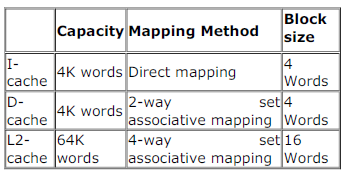The length of the physical address of a word in the main memory is 30 bits. The capacity of the tag memory in the I-cache, D-cache and L2-cache is, respectively,

Solution:

1. I-cache

• Number of blocks in cache = 4K/4 = 210 blocks
• Bits to represent blocks = 10
• Number of words in a block = 4 = 22 words
• Bits to represent words = 2
• tag bits = 30 - (10+2) = 18
• Each block will have it's own tag bits. So total tag bits = 1K x 18 bits.

2. D-cache

• Number of blocks in cache = 4K/4 = 210 blocks
• Number of sets in cache = 210/2 = 29 sets
• Bits to represent sets = 9
• Number of words in a block = 4 = 22 words
• Bits to represent words = 2
• tag bits = 30 - (+2) = 19
• Each block will have it's own tag bits. So total tag bits = 1K x 19 bits.

3. L2 cache

• Number of blocks in cache = 64K/16 = 212 blocks
• Number of sets in cache = 212/4 = 210 sets
• Bits to represent sets = 10
• Number of words in cache = 16 = 24 words
• Bits to represent words = 4
• tag bits = 30 - (10+4) = 16
• Each block will have it's own tag bits. So total tag bits = 212 x 16 bits = 4K x 16 bits

Option A.

QUESTION: 3

### Consider a 4-way set associative cache consisting of 128 lines with a line size of 64 words. The CPU generates a 20-bitaddress of a word in main memory. The number of bits in the TAG, LINE and WORD fields are respectively:

Solution:

Number of sets = cache size/(size of a block * No. of blocks in a set)
= 128 * 64 / (64 * 4) (4 way set associative means 4 blocks in a set)
= 32.
So, number of index (LINE) bits = 5 and number of WORD bits = 6 size cache block (line) size is 64. So, number of TAG
bits = 20 - 6 - 5 = 9.

QUESTION: 4

Consider a 4-way set associative cache consisting of 128 lines with a line size of 64 words. The CPU generates a 20-bitaddress of a word in main memory. The number of bits in the TAG, LINE and WORD fields are respectively:

Solution:

Number of sets = cache size/(size of a block * No. of blocks in a set)
= 128 * 64 / (64 * 4) (4 way set associative means 4 blocks in a set)
= 32.
So, number of index (LINE) bits = 5 and number of WORD bits = 6 size cache block (line) size is 64. So, number of TAG
bits = 20 - 6 - 5 = 9.

QUESTION: 5

Consider a machine with a byte addressable main memory of 216 bytes. Assume that a direct mapped data cache consistingof 32 lines of 64 bytes each is used in the system. A 50 x 50 two-dimensional array of bytes is stored in the main memorystarting from memory location 1100H. Assume that the data cache is initially empty. The complete array is accessed twice.Assume that the contents of the data cache do not change in between the two accesses.How many data misses will occur in total?

Solution:

216 = 64 kb main memory is mapped to 32 lines of 64 bytes. So, number of offset bits = 6 (to identify a byte in a line) and number of indexing bits = 5 (to identify the line).
Size of array = 50* 50 = 2500 B. If array is stored in row-major order (first row, second-row..), and if elements are also accessed in row-major order (or stored and accessed in column-major order), for the first 64 accesses, there will be only 1
cache miss, and for 2500 accesses, there will be 2500/64 = 40 cache misses during the first iteration.
We have 5 index bits and 6 offset bits. So, for 211 (5 + 6 = 11) continuous memory addresses there wont be any cache
conflicts as the least significant bits are offset bits followed by index bits.
So, number of array elements that can be accessed without cache conflict = 2048 (as element size is a byte). The next
452 elements conflict with the first 452 elements. This means 452/64 = 8 cache blocks are replaced. (We used ceil, as
even if one element from a new block is accessed, the whole block must be fetched).
So, during second iteration we incur misses for the first 8 cache blocks as well as for the last 8 cache blocks. So, total data
cache misses across 2 iterations = 40 + 8 + 8 = 56.

QUESTION: 6

Consider a Direct Mapped Cache with 8 cache blocks (numbered 0-7). If the memory block requests are in the following order
3, 5, 2, 8, 0, 63, 9,16, 20, 17, 25, 18, 30, 24, 2, 63, 5, 82,17, 24.
Which of the following memory blocks will not be in the cache at the end of the sequence ?

Solution:

ans is B
cache location (memory block) = block req mod number of cache blocks. Since each block has only one location
(associativity is 1) the last mod 8 request will be in cache (no need of any replacement policy as mapping is direct).
3, 5, 2, 8, 0, 63, 9, 16, 20, 17, 25, 18, 30, 24, 2, 63, 5, 82, 17, 24
Block 0- 8, 0, 16, 24, 24. At end contains 24.

1- 9, 17, 25, 17.
2- 2, 18, 2, 82.
3- 3.
4- 20.
5- 5, 5.
6- 30.
7- 63 63.
So, memory block 18 is not in cache while 3, 20 and 30 are in cache.

QUESTION: 7

For inclusion to hold between two cache levels L1 and L2 in a multi-level cache hierarchy, which of the following are necessary?
I. L1 must be write-through cache
II. L2 must be a write-through cache
III. The associativity of L2 must be greater than that of L1
IV. The L2 cache must be at least as large as the L1 cache

Solution:

1st is not correct as data need not to be exactly same at the same point of time and so write back policy can be used in
this.
2nd is not needed when talking only about L1 and L2.
For 3rd, associativity can be equal.
So only 4th statement is Necessarily true - A choice.

QUESTION: 8

Consider a machine with a byte addressable main memory of bytes. Assume that a direct mapped data cache consistingof 32 lines of 64 bytes each is used in the system. A 50 x 50 two-dimensional array of bytes is stored in the main memorystarting from memory location 1100H. Assume that the data cache is initially empty. The complete array is accessed twice.Assume that the contents of the data cache do not change in between the two accesses.Which of the following lines of the data cache will be replaced by new blocks in accessing the array for the second time?

Solution:

Cache Organization:
We need to find Starting block = 4352B/64B = 68th block in main memory from where array start storing elements.
50*50B =array size = 50*50B/64B =39.0625 blocks needed =approx = 40 blocks
68,69,70....107 block we need = 40 blocks starting block is 68 mod 32 = 4th cache block and after that in sequence they will be accessed.
as shown in below table line no 4 to 11 has been replaced by array in second time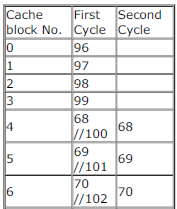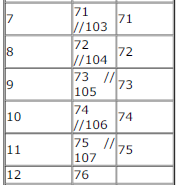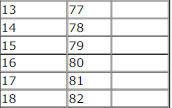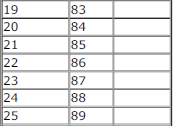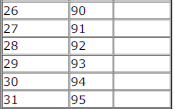QUESTION: 9

Consider a machine with a 2-way set associative data cache of size 64 Kbytes and block size 16 bytes. The cache is managed using 32 bit virtual addresses and the page size is 4 Kbytes. A program to be run on this machine begins as follows:
double ARR;
int i, j;
/*Initialize array ARR to 0.0 */
for(i = 0; i < 1024; i++)
for(j = 0; j < 1024; j++)
ARR[i][j] = 0.0;
The size of double is 8 bytes. Array ARR is located in memory starting at the beginning of virtual page 0xFF000 and stored in row major order. The cache is initially empty and no pre-fetching is done. The only data memory references made by the program are those to array ARR.
The total size of the tags in the cache directory is

Solution:

Number of sets = cache size/ size of a set = 64 KB / (16 B * 2) (two blocks per set)
= 2 K = 211
So, we need 11 bits for set indexing.
Number of WORD bits required = 4 as a cache block consists of 16 bytes and we need 4 bits to address each of them.
So, number of tag bits = 32 - 11 - 4 = 17
Total size of the tag = 17 * Number of cache blocks
= 17 * 211 * 2 (since each set has 2 blocks)
= 68 Kbits answer = option D) 68 Kbits
We use the top 17 bits for tag and the next 11 bits for indexing and next 4 for offset. So, for two addresses to have the same cache index, their 11 address bits after the 4 offset bits from right must be same.
ARR is located at virtual address 0x FF000 000. (FF000 is page address and 000 is page offset). So, index bits are 00000000000
Address of ARR = 0xFF000 + 4 * sizeof (double) = 0xFF000 000 + 4*8 = 0xFF000 020 (32 = 20 in hex) (index bits differ)
Address of ARR = 0xFF000 + 4 * 1024 * sizeof (double) [since we use row major storage] = 0xFF000 000 + 4096*8
= 0xFF000 000 + 0x8000 = 0xFF008 000 ( index bits matches that of ARR  as both read 000 0000 0000)
Address of ARR = 0xFF000 + 5 * sizeof (double) = 0xFF000 000+ 5*8 = 0xFF000 028 (40 = 28 in hex) (index bits
differ)
Address of ARR = 0xFF000 + 5 * 1024 * sizeof (double) [since we use row major storage] = 0xFF000 000 + 5120*8
= 0xFF000 000 + 0xA000 = 0xFF00A 000 (index bits differ)
So, only ARR and ARR have the same cache index.
The inner loop is iterating from 0 to 1023, so consecutive memory locations are accessed in sequence. Since cache block

size is only 16 bytes and our element being double is of size 8 bytes, during a memory access only the next element gets
filled in the cache. i.e.; every alternative memory access is a cache miss giving a hit ratio of 50%. (If loops i and j are
reversed, all accesses will be misses and hit ratio will become 0).

QUESTION: 10

Consider a machine with a 2-way set associative data cache of size 64 Kbytes and block size 16 bytes. The cache is managed using 32 bit virtual addresses and the page size is 4 Kbytes. A program to be run on this machine begins as follows:
double ARR;
int i, j;
/*Initialize array ARR to 0.0 */
for(i = 0; i < 1024; i++)
for(j = 0; j < 1024; j++)
ARR[i][j] = 0.0;
The size of double is 8 bytes. Array ARR is located in memory starting at the beginning of virtual page 0xFF000 and stored in
row major order. The cache is initially empty and no pre-fetching is done. The only data memory references made by the
program are those to array ARR.

Which of the following array elements have the same cache index as ARR?

Solution:

Number of sets = cache size/ size of a set = 64 KB / (16 B * 2) (two blocks per set) = 2 K = 211
So, we need 11 bits for set indexing.

Number of WORD bits required = 4 as a cache block consists of 16 bytes and we need 4 bits to address each of them.
So, number of tag bits = 32 - 11 - 4 = 17
Total size of the tag = 17 * Number of cache blocks = 17 * 211 * 2 (since each set has 2 blocks)
= 68 KB
We use the top 17 bits for tag and the next 11 bits for indexing and next 4 for offset. So, for two addresses to have the same cache index, their 11 address bits after the 4 offset bits from right must be same.
ARR is located at virtual address 0x FF000 000. (FF000 is page address and 000 is page offset). So, index bits are 00000000000
Address of ARR = 0xFF000 + 4 * sizeof (double) = 0xFF000 000 + 4*8 = 0xFF000 020 (32 = 20 in hex) (index bits
differ)
Address of ARR = 0xFF000 + 4 * 1024 * sizeof (double) [since we use row major storage] = 0xFF000 000 + 4096*8 = 0xFF000 000 + 0x8000 = 0xFF008 000 ( index bits matches that of ARR  as both read 000 0000 0000)
Address of ARR = 0xFF000 + 5 * sizeof (double) = 0xFF000 000+ 5*8 = 0xFF000 028 (40 = 28 in hex) (index bits differ)
Address of ARR = 0xFF000 + 5 * 1024 * sizeof (double) [since we use row major storage] = 0xFF000 000 + 5120*8 = 0xFF000 000 + 0xA000 = 0xFF00A 000 (index bits differ)
So, only ARR and ARR have the same cache index.
The inner loop is iterating from 0 to 1023, so consecutive memory locations are accessed in sequence. Since cache block size is only 16 bytes and our element being double is of size 8 bytes, during a memory access only the next element gets
filled in the cache. i.e.; every alternative memory access is a cache miss giving a hit ratio of 50%. (If loops i and j are reversed, all accesses will be misses and hit ratio will become 0).

QUESTION: 11

Consider a machine with a 2-way set associative data cache of size 64 Kbytes and block size 16 bytes. The cache is
managed using 32 bit virtual addresses and the page size is 4 Kbytes. A program to be run on this machine begins as
follows:
double ARR;
int i, j;
/*Initialize array ARR to 0.0 */
for(i = 0; i < 1024; i++)
for(j = 0; j < 1024; j++)
ARR[i][j] = 0.0;
The size of double is 8 bytes. Array ARR is located in memory starting at the beginning of virtual page 0xFF000 and stored in
row major order. The cache is initially empty and no pre-fetching is done. The only data memory references made by the
program are those to array ARR.
The cache hit ratio for this initialization loop is

Solution:

block size=16B and one element=8B.so in one block 2 element will be stored.
for 1024*1024 element num of block required=1024*1024/2 =2^19 blocks required.
in one block first element will be a miss and second one is hit(since we are transferring two unit at a time)
=>hit ratio=total hit/total reference
=2^19/2^20
=1/2=0.5
=0.5*100=50%

QUESTION: 12

Consider a machine with a 2-way set associative data cache of size 64 Kbytes and block size 16 bytes. The cache is
managed using 32 bit virtual addresses and the page size is 4 Kbytes. A program to be run on this machine begins as
follows:
double ARR;
int i, j;
/*Initialize array ARR to 0.0 */
for(i = 0; i < 1024; i++)
for(j = 0; j < 1024; j++)
ARR[i][j] = 0.0;
The size of double is 8 bytes. Array ARR is located in memory starting at the beginning of virtual page 0xFF000 and stored in
row major order. The cache is initially empty and no pre-fetching is done. The only data memory references made by the
program are those to array ARR.
The cache hit ratio for this initialization loop is

Solution:

block size=16B and one element=8B.so in one block 2 element will be stored.
for 1024*1024 element num of block required=1024*1024/2 =2^19 blocks required.
in one block first element will be a miss and second one is hit(since we are transferring two unit at a time)
=>hit ratio=total hit/total reference
=2^19/2^20
=1/2=0.5
=0.5*100=50%

QUESTION: 13

Consider a machine with a 2-way set associative data cache of size 64 Kbytes and block size 16 bytes. The cache is
managed using 32 bit virtual addresses and the page size is 4 Kbytes. A program to be run on this machine begins as
follows:
double ARR;
int i, j;
/*Initialize array ARR to 0.0 */
for(i = 0; i < 1024; i++)
for(j = 0; j < 1024; j++)
ARR[i][j] = 0.0;
The size of double is 8 bytes. Array ARR is located in memory starting at the beginning of virtual page 0xFF000 and stored in
row major order. The cache is initially empty and no pre-fetching is done. The only data memory references made by the
program are those to array ARR.
The cache hit ratio for this initialization loop is

Solution:

block size=16B and one element=8B.so in one block 2 element will be stored.
for 1024*1024 element num of block required=1024*1024/2 =2^19 blocks required.
in one block first element will be a miss and second one is hit(since we are transferring two unit at a time)
=>hit ratio=total hit/total reference
=2^19/2^20
=1/2=0.5
=0.5*100=50%

QUESTION: 14

Consider a computer with a 4-ways set-associative mapped cache of the following characteristics: a total of 1 MB of main memory, a word size of 1 byte, a block size of 128 words and a cache size of 8 KB.
The number of bits in the TAG, SET and WORD fields, respectively are:

Solution:

Number of cache blocks = 8KB/(128*1) = 64
Number of sets in cache = Number of cache blocks/ 4 (4-way set)
= 64 / 4 = 16
So, number of SET bits required = 4 (as 24 = 16, and with 4 bits we can get 16 possible outputs) We can now straight away choose (D) as answer but for confirmation can proceed further.
Since, only physical memory information is given we can assume cache is physically tagged (which is anyway the common
case even in case of virtual memory). So, we can divide the physical memory into 16 regions so that, each set maps into only its assigned region. So, size of a region a set can address = 1MB/16 = 216 Bytes = 21 6 /128 = 29 cache blocks (as cache block size is 128 words = 128 bytes). So, when an access comes to an cache entry, it must be able to determine which out of the 29 possible physical block it is. In short it needs 9 bits for TAG.
Now, cache block size is 128 words and so to identify a word we need 7 bits for WORD.

QUESTION: 15

Consider a computer with a 4-ways set-associative mapped cache of the following characteristics: a total of 1 MB of main memory, a word size of 1 byte, a block size of 128 words and a cache size of 8 KB.
While accessing the memory location 0C795H by the CPU, the contents of the TAG field of the corresponding cache line is

Solution:

we have 16 sets in cache and correspondingly 16 regions in
physical memory to which each set is mapped. Now, WORD bit size is 7 as we need 7 bits to address 128 possible words in
a cache block. So, the lowest 7 bits of 0C795H will be used for this giving us the remaining bits as
0000 1100 0111 1
Of these bits, the lower 4 are used for addressing the 16 possible sets, giving us the tag bits: 0000 1100 0 in (A) choice.

QUESTION: 16

Consider a 4-way set associative cache (initially empty) with total 16 cache blocks. The main memory consists of 256 blocksand the request for memory blocks are in the following order:0, 255, 1, 4, 3, 8, 133, 159, 216, 129, 63, 8, 48, 32, 73, 92, 155.Which one of the following memory block will NOT be in cache if LRU replacement policy is used?

Solution:

16 blocks and sets with 4 blocks each means there are 4 sets. So, the lower 2 bits are used for getting a set. And 4 way associative means in a set only the last 4 cache accesses can be stored.
0, 255, 1, 4, 3, 8, 133, 159, 216, 129, 63, 8, 48, 32, 73, 92, 155
Mod 4 gives
0, 3, 1, 0, 3, 0, 1, 3, 0, 1, 3, 0, 0, 0, 1, 0, 3
Now for each of 0..3, the last 4 accesses will be in cache. So, {92, 32, 48, 8}, {155, 63, 159, 3}, {73, 129, 133, 1} and
{} will be in cache. So, the missing element from choice is 216.

QUESTION: 17

A computer system has an L1 cache, an L2 cache, and a main memory unit connected as shown below. The block size in L1cache is 4 words. The block size in L2 cache is 16 words. The memory access times are 2 nanoseconds, 20 nano seconds and 200 nano seconds for L1 cache, L2 cache and the main memory unit respectively.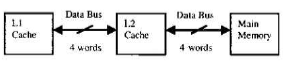When there is a miss in L1 cache and a hit in L2 cache, a block is transferred from L2 cache to L1 cache. What is the timetaken for this transfer?

Solution:

Ideally the answer should be 20 ns as it is the time to transfer a block from L2 to L1 and this time only is asked in
question. But there is confusion regarding access time of L2 as this means the time to read data from L2 till CPU but here
we need the time till L1 only. So, I assume the following is what is meant by the question.
A block is transferred from L2 to L1. And L1 block size being 4 words (since L1 is requesting we need to consider L1 block
size and not L2 block size) and data width being 4 bytes, it requires one L2 access (for read) and one L1 access (for store).
So, time = 20+2 = 22 ns.

QUESTION: 18

A computer system has an L1 cache, an L2 cache, and a main memory unit connected as shown below. The block size in L1cache is 4 words. The block size in L2 cache is 16 words. The memory access times are 2 nanoseconds, 20 nano seconds and 200 nano seconds for L1 cache, L2 cache and the main memory unit respectively.When there is a miss in L1 cache and a hit in L2 cache, a block is transferred from L2 cache to L1 cache. What is the timetaken for this transfer?

Solution:

Ideally the answer should be 20 ns as it is the time to transfer a block from L2 to L1 and this time only is asked in
question. But there is confusion regarding access time of L2 as this means the time to read data from L2 till CPU but here
we need the time till L1 only. So, I assume the following is what is meant by the question.
A block is transferred from L2 to L1. And L1 block size being 4 words (since L1 is requesting we need to consider L1 block
size and not L2 block size) and data width being 4 bytes, it requires one L2 access (for read) and one L1 access (for store).
So, time = 20+2 = 22 ns.

QUESTION: 19

A computer system has an L1 cache, an L2 cache, and a main memory unit connected as shown below. The block size in L1cache is 4 words. The block size in L2 cache is 16 words. The memory access times are 2 nanoseconds, 20 nano seconds and 200 nanoseconds for L1 cache, L2 cache and the main memory unit respectively.When there is a miss in L1 cache and a hit in L2 cache, a block is transferred from L2 cache to L1 cache. What is the timetaken for this transfer?

Solution:

The transfer time should be 4*200 + 20 = 820 ns. But this is not in option. So, I assume the following is what is meant by the question.
L2 block size being 16 words and data width between memory and L2 being 4 words, we require 4 memory accesses(for read) and 4 L2 accesses (for store). Now, we need to send the requested block to L1 which would require one more L2 access (for read) and one L1 access (for store). So, total time
= 4 * (200 + 20) + (20 + 2)
= 880 + 22
= 902 ns

QUESTION: 20

A computer system has an L1 cache, an L2 cache, and a main memory unit connected as shown below. The block size in L1cache is 4 words. The block size in L2 cache is 16 words. The memory access times are 2 nanoseconds, 20 nano seconds and 200 nano seconds for L1 cache, L2 cache and the main memory unit respectively.When there is a miss in L1 cache and a hit in L2 cache, a block is transferred from L2 cache to L1 cache. What is the timetaken for this transfer?

Solution:

Ideally the answer should be 20 ns as it is the time to transfer a block from L2 to L1 and this time only is asked in
question. But there is confusion regarding access time of L2 as this means the time to read data from L2 till CPU but here
we need the time till L1 only. So, I assume the following is what is meant by the question.
A block is transferred from L2 to L1. And L1 block size being 4 words (since L1 is requesting we need to consider L1 block
size and not L2 block size) and data width being 4 bytes, it requires one L2 access (for read) and one L1 access (for store).
So, time = 20+2 = 22 ns.

QUESTION: 21

An 8KB direct-mapped write-back cache is organized as multiple blocks, each size of 32-bytes. The processor generates 32- bit addresses. The cache controller contains the tag information for each cache block comprising of the following.
1 valid bit
1 modified bit
As many bits as the minimum needed to identify the memory block mapped in the cache.
What is the total size of memory needed at the cache controller to store meta-data (tags) for the cache?

Solution:

Number of cache blocks = cache size / size of a block
=8 KB/32 B
=256
So, we need 8 bits for indexing the 256 blocks of the cache. And since a block is 32 bytes we need 5 WORD bits to address
each byte. So, out of the remaining 19 bits (32 - 8 - 5) should be tag bits.
So, a tag entry size = 19 + 1(valid bit) + 1(modified bit) = 21 bits.
Total size of metadata = 21 * Number of cache blocks
= 21 * 256
= 5376 bits

QUESTION: 22

A computer has a
256
KByte, 4-way set associative, write back data cache with block size of
32 . The processor sends
Bytes addresses to the cache controller. Each cache tag directory entry contains, in addition to
32
2 valid bits,
1 modified bit and
1 replacement bit.
The number of bits in the tag field of an address is

Solution:

Total cache size = 256 KB
Cache block size = 32 Bytes
So, number of cache entries = 256 K / 32 = 8 K
Number of sets in cache = 8 K/4 = 2 K as cache is 4-way associative.
So, log(2048) = 11 bits are needed for accessing a set. Inside a set we need to identify the cache entry.
No. of memory block possible = Memory size/Cache block size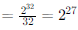So, no. of memory block that can go to a single cache set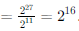So, we need 16 tag bits along with each cache entry to identify which of the possible 216 blocks is being mapped there.

QUESTION: 23

A computer has a 256 - KByte, 4-way set associative, write back data cache with block size of 32 Bytes. The processor sends 32 bit addresses to the cache controller. Each cache tag directory entry contains, in addition to address tag, 2 valid bits, 1 modified bit and 1 replacement bit. The size of the cache tag directory is

Solution:

Total cache size = 256 KB
Cache block size = 32 Bytes

So, number of cache entries = 256 K / 32 = 8 K
Number of sets in cache = 8 K/4 = 2 K as cache is 4-way associative.
So, log(2048) = 11 bits are needed for accessing a set. Inside a set we need to identify the cache entry.
Total number of distinct cache entries = 232/cache entry size = 232/32 = 227
Out of this 227, each set will be getting only 227/211 = 216 possible distinct cache entries as we use the first 11 bits to
identify a set. So, we need 16 bits to identify a cache entry in a set, which is the number of bits in the tag field.
Size of cache tag directory = Size of tag entry * Number of tag entries
= 16 +(2+1+1) bits (2 valid, 1 modified, 1 replacement as given in question) * 8 K
= 20 * 8 = 160 Kbits
Not needed for this question, still:
Valid bit: Tells if the memory referenced by the cache entry is valid. Initially, when a process is loaded all entries are
invalid. Only when a page is loaded, its entry becomes valid.
Modified bit: When processor writes to a cache location its modified bit is made 1. This information is used when a cache
entry is replaced- entry 0 means no update to main memory needed. Entry 1 means an update is needed.

QUESTION: 24

In a -way set associative cache, the cache is divided into sets, each of which consists of lines. The lines of a set are placed in sequence one after another. The lines in set are sequenced before the lines in set . The main memory blocks are numbered 0 onwards. The main memory block numbered must be mapped to any one of the cache lines from)

Solution:

Number of sets in cache = v. The question gives a sequencing for the cache lines. For set 0, the cache lines are numbered
0, 1, .., k-1. Now for set 1, the cache lines are numbered k, k+1,... k+k-1 and so on. So, main memory block j will be
mapped to set (j mod v), which will be any one of the cache lines from (j mod v) * k to (j mod v) * k + (k-1).
(Associativity plays no role in mapping- k-way associativity means there are k spaces for a block and hence reduces the
chances of replacement.)

QUESTION: 25

An access sequence of cache block addresses is of length N and contains n unique block addresses. The number of unique block addresses between two consecutive accesses to the same block address is bounded above by . What is the miss ratio if the access sequence is passed through a cache of associativity A ≥ Kexercising least-recently-used replacement policy?

Solution:

There are N accesses to cache.
Out of these n are unique block addresses.
Now, we need to find the number of misses. (min. n misses are guaranteed whatever be the access sequence due to n unique block addresses)
We are given that between two consecutive accesses to the same block, there can be only k unique block addresses. So,
for a block to get replaced we can assume that all the next k block addresses goes to the same set (given cache is setassociative)
which will be the worst case scenario (they may also go to a different set but then there is lesser chance of a
replacement). Now, if associativity size is >= k, and if we use LRU (Least Recently Used) replacement policy, we can guarantee that these k accesses won't throw out our previously accessed cache entry (for that we need at least k
accesses). So, this means we are at the best-cache scenario for cache replacement -- out of N accesses we miss only n (which are unique and can not be helped from getting missed and there is no block replacement in cache). So, miss ratio is n/N.
PS: In question it is given "bounded above by k", which should mean k unique block accesses as k is an integer, but to ensure no replacement this must be 'k-1'. Guess, a mistake in question.

QUESTION: 26

In designing a computer's cache system, the cache block (or cache line) size is an important parameter. Which one of thefollowing statements is correct in this context?

Solution:

(A) A smaller block size means during a memory access only a smaller part of near by addresses are brought to cachemeaning
spatial locality is reduced.
(B) A smaller block size means more number of blocks (assuming cache size constant)and hence we need more cache tag
bits to identify the correct block. So, cache tag becomes bigger.
(C) A smaller block size implying larger cache tag is true, but this can't lower cache hit time in any way.
(D) A smaller block size incurs a lower cache miss penalty. This is because during a cache miss, an entire cache block is fetched from next lower level of memory. So, a smaller block size means only a smaller amount of data needs to be fetched and hence reduces the miss penalty (Cache block size can go til the size of data bus to the next level of memory, and beyond this only increasing the cache block size increases the cache miss penalty).

QUESTION: 27

If the associativity of a processor cache is doubled while keeping the capacity and block size unchanged, which one of thefollowing is guaranteed to be NOT affected?

Solution:

If associativity is doubled, keeping the capacity and block size constant, then the number of sets gets halved. So, width of set index decoder can surely decrease - (B) is false.
Width of way-selection multiplexer must be increased as we have to double the ways to choose from- (C) is false
As the number of sets gets decreased, the number of possible cache block entries that a set maps to gets increased. So,
we need more tag bits to identify the correct entry. So, (A) is also false.
(D) is the correct answer- main memory data bus has nothing to do with cache associativity- this can be answered without
even looking at other options.

QUESTION: 28

A 4-way set-associative cache memory unit with a capacity of 16 KB is built using a block size of 8 words. The word length is 32 bits. The size of the physical address space is 4 GB. The number of bits for the TAG field is ____

Solution:

Number of sets = cache size / sizeof a set
Size of a set = blocksize * no. of blocks in a set
= 8 words * 4 (4-way set-associative)
= 8*4*4 (since a word is 32 bits = 4 bytes)
= 128 bytes.
So, number of sets = 16 KB / (128 B) = 128
Now, we can divide the physical address space equally between these 128 sets. So, the number of bytes each set can access
= 4 GB / 128
= 32 MB
= 32/4 = 8 M words = 1 M blocks. (220 blocks)
So, we need 20 tag bits to identify these 220 blocks.

QUESTION: 29

The memory access time is 1 nanosecond for a read operation with a hit in cache, 5 nanoseconds for a read operation with a miss in cache, 2 nanoseconds for a write operation with a hit in cache and 10 nanoseconds for a write operation with a miss in cache. Execution of a sequence of instructions involves 100 instruction fetch operations, 60 memory operand read
operations and 40 memory operand write operations. The cache hit-ratio is 0.9. The average memory access time (in nanoseconds) in executing the sequence of instructions is ______.

Solution:

Fetch is also a memory read operation. Avg access time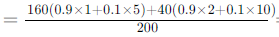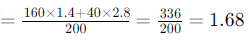*Answer can only contain numeric values
QUESTION: 30

Assume that for a certain processor, a read request takes 50 nanoseconds on a cache miss and 5 nanoseconds on a cache hit. Suppose while running a program, it was observed that 80% of the processor's read requests result in a cache hit. The average read access time in nanoseconds is _____.

Solution: